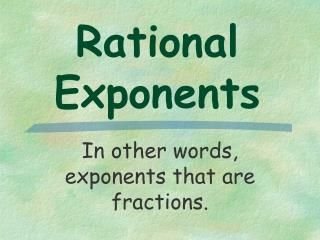DownloadDownload PresentationRational Exponents

# Rational Exponents

Télécharger la présentation## Rational Exponents

- - - - - - - - - - - - - - - - - - - - - - - - - - - E N D - - - - - - - - - - - - - - - - - - - - - - - - - - -
##### Presentation Transcript

1. Rational Exponents In other words, exponents that are fractions.

2. Definition of For any real number b and any integer n > 1, except when b < 0 and n is even

3. Examples:

4. Examples:

5. Economists refer to inflation as increases in the average cost of purchases. The formula C = c(1 + r)n can be used to predict the cost of consumer items at some projected time. In this formula C represents the projected cost of the item at the given annual inflation rate, c the present cost of the item and r is the rate of inflation (in decimal form), and n is the number of years for the projection. Suppose a gallon of milk costs \$2.69 now. How much would the price increase in 6 months with an inflation rate of 5.3%?

6. Step 1: Identify the known values Formula C = c(1 + r)n c = \$2.69 present cost of the item r = 0.053 rate of inflation (in decimal form) n = 1/2 # of years for the projection

7. Step 2: Find the value for C Formula C = c(1 + r)n C = 2.69(1 + 0.053)1/2 C = 2.69(1.053)1/2 C = \$2.76 Answer the question How much would the price increase? \$2.76-\$2.69 = \$0.07 or 7¢

8. Definition of Rational Exponents For any nonzero number b and any integers m and n with n > 1, except when b < 0 and n is even

9. Examples:

10. Simplifying Expressions • No negative exponents • No fractional exponents in the denominator • No complex fractions (fraction within a fraction) • The index of any remaining radical is the least possible number

11. Examples: Simplify each expression Rewrite as a radical Get a common denominator - this is going to be our index

12. Examples: Simplify each expression Remember we add exponents

13. Examples: Simplify each expression To rationalize the denominator we want an integer exponent

14. Examples: Simplify each expression To rationalize the denominator we want an integer exponent

15. Examples: Simplify each expression

16. Examples: Simplify each expression

17. Examples: Simplify each expression Multiply by conjugate and use FOIL

18. Examples: Simplify each expression

19. Examples: Simplify each expression

20. Examples: Simplify each expression

21. Examples: Simplify each expression